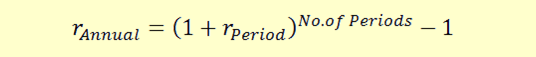# How to Calculate Annualized Returns

When we make investments, we invest our money in different assets and earn returns for different periods of time. For example, an investment in a short-term Treasury bill will be for 3 months. We may invest in a stock and exit after a week for a few days. For the purpose of making the returns on these different investments comparable, we need to annualize the returns. So, all daily, weekly, monthly, or quarterly returns will be converted to annualized returns. The process for annualizing the returns is as follows:

The basic idea is to compound the returns to an annual period. So, if we have monthly returns, we know that there are 12 months in the year, similarly there are 52 weeks, 4 quarters, and 365 days. We compound our returns by the number of periods in the whole year.Let’s take a few examples to understand this.

Example 1: Quarterly Returns

Let’s say we have 5% quarterly returns. Since there are four quarters in a year, the annual returns will be:

Annual returns = (1+0.05)4 – 1 = 21.55%

Example 2: Monthly Returns

Let’s say we have 2% monthly returns. Since there are 12 months in a year, the annual returns will be: